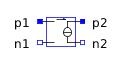Cylindrical Geometry - MapleSim Help

Cylindrical Geometry

Display a cylinder in your 3-D modelDescription The length of the cylinder is displayed between the points at which the component are connected in the model workspace. If you connect this shape to the frames of a single component,a cylinder shape is displayed over the implicit geometry that represents the component in the 3-D workspace.If you connect this component to the frames of two different modeling components,the cylinder shape spans multiple components.If the Partial Cylinder option is used, a third frame (frame_c) is used to define the orientation,The parameter $\mathrm{θ}$ defines the covered angle (right-hand rule)Connections

 Name Condition Description Modelica ID frame_a Defines the location of the first edge for the cylinder length. frame_a frame_b Defines the location of the second edge for the cylinder length. frame_b frame_c Defines the reference orientation of the partial cylinder. frame_c inputColor Dynamic Color = true Defines the shape color. Must be connected to the output of a Color Blend component. inputColor r_in Use inputs = true 2-vector signal input defining the inner and outer radii of the cylinder. r_in theta_in Use inputs = true Use partial = true 2-vector signal input defining the start and end angles of the cross-section. The angles are defined w.r.t. vector from frame_a to frame_c. theta_inParameters

 Name Default Units Condition Description Modelica ID outer radius 0.1 m Use inputs = false Outer radius of the cylinder. radius inner radius 0 m Use inputs = false Inner radius of the cylinder. innerRadius Partial CylinderWhen checked (true) a third frame (frame_c) is enabled to define the reference orientation of the partial cylinder. usePartial $\mathrm{\theta }$ 360. deg Partial Cylinder = true Use inputs = false Angle of display arc. theta color red Dynamic Color = false Color of the cylinder. For information on using the color selection tools, see Selecting Colors. color Dynamic ColorSelect this option to enable the Dynamic Color port. With the Dynamic Color port enabled, you can use the Color Blend component to change color throughout the simulation. isInputColor TransparentSelect this option to make the geometry component transparent. transparent Use InputsWhen checked (true), inner/outer radii (as well as start and end angles, if partial) are given via real input signals. useInputSee Also